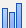The Bar elementshows a bar chart of summary statistic values of one or more variables across the values or categories of one or more stratifying variables. The graph can display a single variable or multiple variables of any type arranged in the X and Y zones.
 • If you add a single categorical variable, then each bar shows the count of observations in the corresponding level of the variable.
 • If you add multiple variables in each zone, multiple bars are plotted for each value or combination of levels of the stratifying variables.
 • Integer values are assigned based on the Value Ordering column property or the default value ordering.
 • These integer values range from 0 (highest level in value ordering) to the number of levels minus one (lowest value in value ordering).
 • These integer values are used in calculating the Summary Statistic.
Figure 2.31 Bar Options
First Stack—Start on the top row and fill it before moving to the next row.
Smallest Stack—Place each bar at the stack that is currently the smallest.
Separate Stack—Make a stack called Other where all secondary bars appear.
Bar color—Applies the same color as the primary bar color.
Grays—Applies random shades of light gray.
 – Show or hide the elements corresponding to a variable in a zone.
 – Add or remove the effect of applying the Color, Size, Shape, or Freq variable to the variable in the zone.
Tip: If you have multiple graphs, you can color or size each graph by different variables. Drag a second variable to the Color or Size zone, and drop it in a corner. In the Variables option, select the specific color or size variable to apply to each graph.
 • The values of the summary statistic are calculated for each of the merged variables.
 • A rectangle is plotted with bounds equal to the summary statistic values for the first two of variables, as indicated in the legend.
 • Lines are plotted at the values of the summary statistic for the other variables.
 • The values of the summary statistic are calculated for each of the merged variables.
 • A line with boundaries is plotted with bounds equal to the summary statistic values for the first two of variables, as indicated in the legend.
 • A circle is plotted at the overall values of the summary statistic for the other variables.
 • The values of the summary statistic are calculated for each of the merged variables.
 • A line segment connecting the summary statistic values for two of the variables, as indicated in the legend.
 • Bars are plotted at right angles to the line segment at the remaining values of the summary statistic.
 • The values of the summary statistic are calculated for each of the merged variables.
 • An outlier box plot is constructed for these values.
 • The values of the summary statistic are calculated for each of the merged variables.
 • A bar is plotted at each of these values.

Help created on 7/12/2018